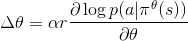# Probability distributions - torch.distributions

distributions 统计分布包中含有可自定义参数的概率分布和采样函数.probs = policy_network(state)
# NOTE: 等同于多项式分布
m = Categorical(probs)
action = m.sample()
next_state, reward = env.step(action)
loss = -m.log_prob(action) * reward
loss.backward()


## Distribution (概率分布)

class torch.distributions.Distribution


Distribution是概率分布的抽象基类.

log_prob(value)


sample()


sample_n(n)


## Bernoulli (伯努利分布)

class torch.distributions.Bernoulli(probs)


>>> m = Bernoulli(torch.Tensor([0.3]))
>>> m.sample()  # 30% chance 1; 70% chance 0
0.0
[torch.FloatTensor of size 1]


## Categorical (类别分布)

class torch.distributions.Categorical(probs)


>>> m = Categorical(torch.Tensor([ 0.25, 0.25, 0.25, 0.25 ]))
>>> m.sample()  # equal probability of 0, 1, 2, 3
3
[torch.LongTensor of size 1]


## Normal (正态分布)

class torch.distributions.Normal(mean, std)


>>> m = Normal(torch.Tensor([0.0]), torch.Tensor([1.0]))
>>> m.sample()  # normally distributed with mean=0 and stddev=1
0.1046
[torch.FloatTensor of size 1]


• mean (float 或 Tensor 或 Variable) – 分布的均值
• std (float 或 Tensor 或 Variable) – 分布的标准差